#### Cournot Duopoly, Cournot-Nash Equilibrium and Generalization to Oligopoly

Cournot Duopoly (Augustine A. Cournot, 1838)

1) Assumptions:

* λi = dQ-i/dqi =  0
* Each firm determines products that maximize their profits taking the products of rivals’ constant.
* Market demand is P = a − Q ( a > 0) and Q = q1 + q2 (Q is the total quantity of spring water sold in the market per unit of time).
* For simplicity, MC = AC = c.

2) Model:

* Firm 2 assumes that firm 1 is producing q1 and it produces q2 as the best response to q1.
* So the economic profit for firm 2 is as follows,

π2 = (P − c)q2 = (a − c − q1 − q2)q2 .

(Δπ2/Δq2) = a-c-q1-2q2= 0   (i). From (i), we get the best response of firm 2 to q1 of firm 1,

q2 = ((a- c- q1)/2)       (ii): Reaction Curve of firm 2

* In the same way we get reaction curve of firm 1.

q1= ((a- c- q2)/2)     (iii): Reaction Curve of firm 1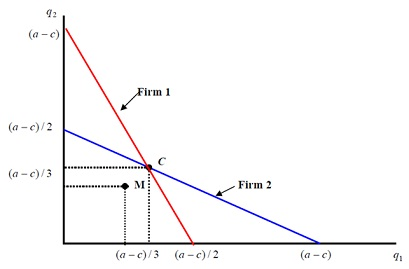Cournot-Nash Equilibrium: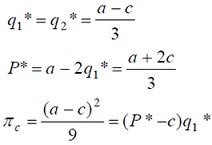* Suppose firm 1 and firm 2 decide to make a Cartel and act as collective monopolist. subsequently what are the profits of two firms?

As well as what is the monopolistic product? (MR = MC)

Given demand curve is P = a − Q . TR = PQ = (a − Q)Q. AndMR = a − 2Q, and MC = c

∴ MR = a − 2Q = c = MC. So Q m (a- c) /2

If these two firms produce the same quantities, then q1=q2= (a-c)/4.

And Pm = (a+c)/2, πm= (a-c)2/8 , which is bigger than profit at point C (πc = (a-c)2/9).

* If the two firms make a Cartel and determine their quantities cooperatively, then their economic profits are higher than those of Cournot-Nash equilibrium. Therefore, there is a good reason and motive to cartelize or to collude.

* However why do we call C as an equilibrium point not M? Or, why is not M is an equilibrium? For the reason that there is another motive to cheat by increasing quantity to earn more profit at point M.

* If firm 1 produces q 1 = (a-c)/ 4 and keeps the Cartel but firm 2 does not, then the profit function of firm 2 is as follows: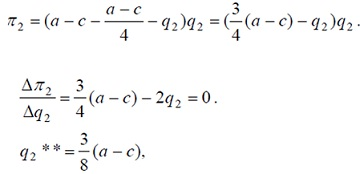This maximizes economic profit of firm 2. Hence, firm 2 breaks the cartel and produces more. Market price of firm 2’s products is P 2 = (3a + 5c)/8 and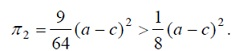* Firm 2 has a motive to cheat firm 1. Vice versa. Therefore one-shot cartel is unstable.

* Cartel is simple to collapse. But, if the cartel is made among few firms for relatively long periods, then it can be sustained longer with efficient and severe punishment or penalty on the violators.

Generalization to Oligopoly: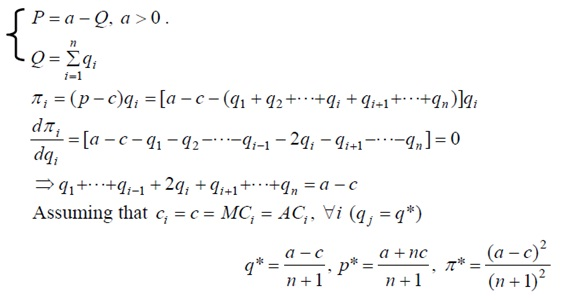If n = 2  we get the similar results in Cournot Duopoly.

Latest technology based Microeconomics Online Tutoring Assistance

Tutors, at the www.tutorsglobe.com, take pledge to provide full satisfaction and assurance in Microeconomics help via online tutoring. Students are getting 100% satisfaction by online tutors across the globe. Here you can get homework help for Microeconomics, project ideas and tutorials. We provide email based Microeconomics help. You can join us to ask queries 24x7 with live, experienced and qualified online tutors specialized in Microeconomics. Through Online Tutoring, you would be able to complete your homework or assignments at your home. Tutors at the TutorsGlobe are committed to provide the best quality online tutoring assistance for Microeconomics Homework help and assignment help services. They use their experience, as they have solved thousands of the Microeconomics assignments, which may help you to solve your complex issues of Microeconomics. TutorsGlobe assure for the best quality compliance to your homework. Compromise with quality is not in our dictionary. If we feel that we are not able to provide the homework help as per the deadline or given instruction by the student, we refund the money of the student without any delay.